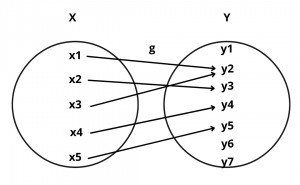# One One Function (Injection) – Definition and Examples

Here you will learn what is one one function with definition and examples.

Let’s begin –

## One One Function Definition

Definition : A function f : A $$\rightarrow$$ B is said to be a one-one function or an injection if different elements of A have different images in B.

Thus, f : A $$\rightarrow$$ B is one-one

$$\iff$$  a $$\ne$$ b  $$\implies$$  f(a) $$\ne$$ f(b) for all a, b $$\in$$ A

$$\iff$$  f(a) = f(b)  $$\implies$$  a = b for all a, b $$\in$$ A

Example : Let f : A $$\rightarrow$$ B and g : X $$\rightarrow$$ Y be two functions represented by the following diagrams :Solution : Clearly, f : A $$\rightarrow$$ B is a one one function. But, g : X $$\rightarrow$$ Y is not one-one because two different elements x1 and x3 have the same image under function g.

Example : Let A = {1, 2, 3, 4}, B = {1, 2, 3, 4, 5, 6} and f : A $$\rightarrow$$ B be a function defined by f(x) = x + 2 for all x $$\in$$ A.

Solution : We observe that f as a set of ordered pairs can be written as f = {(1, 3), (2, 4), (3, 5), (4, 6)}

Clearly, different elements in A have different images under function f.

So, f : A $$\rightarrow$$ B is an injection or one-one.

## Algorithm to Check for Injection

1).  Take two arbitrary elements x, y (say) in the domain of f.

2).  Put f(x) = f(y)

3).  Solve f(x) = f(y). If it gives x = y only, then f : A $$\rightarrow$$ B is a one-one function (or an injection) otherwise not.

## Number of One One Function

If A and B are two sets having m and n elements respectively such that m $$\le$$ n, then the total number of one-one functions from A to B is

$$^nC_m \times m!$$

where m! is m factorial.